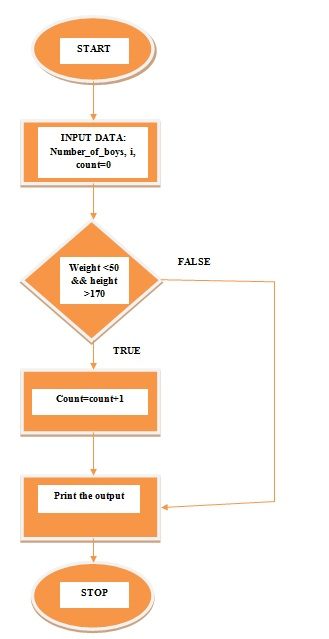## IF STATEMENT  IN C

if-statement is a decision-making statement (in real life, we need to take some decision, these decisions decide what should we do next, the same condition for programming which decides what block of code will execute). When the condition in if-statement is true then the block of code will execute and condition in if-statement is false then the block of code will not execute.

Syntax:

if (condition)
{

//Block of code

}

### FLOW CHART of if Statement:

If curly braces ({ ) is not provided after if statement then only first statement will execute and rest of the statement will not execute.

### Algorithm

Step1:  Start the program.
Step2:  Enter input data a =14, b=13.
Step3: Write if statement with condition (a>b).
Step4:  When the condition (a>b)is true then the block of code will execute. When the condition (a>b) is false then go to Step5.
Step5: print the output.
Step6: Finish the program.

### FLOW CHART OF LARGEST NUMBER PROGRAMFlow chart of the largest number using if statement

### PROGRAM

#include <stdio.h>
int main()
{
int a =14, b = 13;
if (a > b)
{
printf ("largest number is=%d\n", a);
}
return 0;
}

OutputThe output of the largest number program

### Algorithm

Step1: Start the program.
Step2: Input data number_of_boys, i, count=0, weight and height.
Step3: Write condition in if statement (weight should be less than 50 kg and height should be greater than 170 cm).
Step4: When if the condition is true then the number of count of boys will increase by one. When the condition is false then goto Step5.
Step5: Print the output.
Step6: Stop the program.

### FLOW CHARTFlow Chart using if statement

### PROGRAM

#include <stdio.h>
int main()
{
int number_of_boys, i, count = 0;
float weight, height;
printf ("Enter number of boys\n");
scanf ("%d", &number_of_boys);
printf ("Entered number_of_boys are %d\n", number_of_boys);

printf ("Enter number of weight and height");

for (i =1;i<=number_of_boys; i++)
{
scanf ("%f%f", &weight, &height);

if (weight < 50 && height >170)
{
count ++;
}
}
printf ("Number of boys with weight less than 50kg and height greater than 170cm %d\n", count);
return 0;
}

OutputThe output of a program using if statement